# Steklov function

for an integrable functionon a bounded segmentThe function(*)

Functions of the form (*), as well as the iteratively defined functions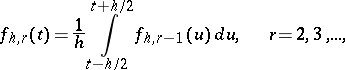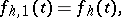were first introduced in 1907 by V.A. Steklov (see ) in solving the problem of expanding a given function into a series of eigenfunctions. The Steklov function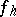has derivativealmost everywhere. Ifis uniformly continuous on the whole real axis, then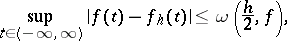where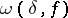is the modulus of continuity of. Similar inequalities hold in the metric of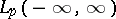, provided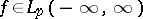.